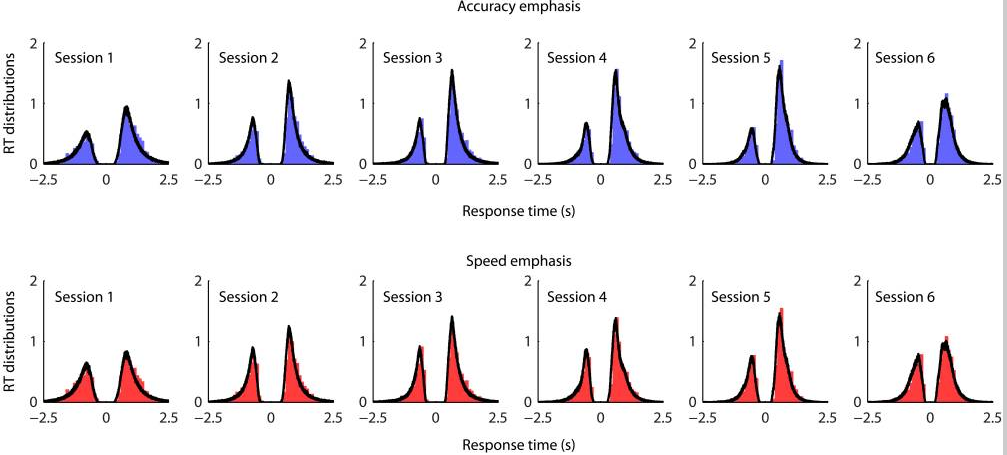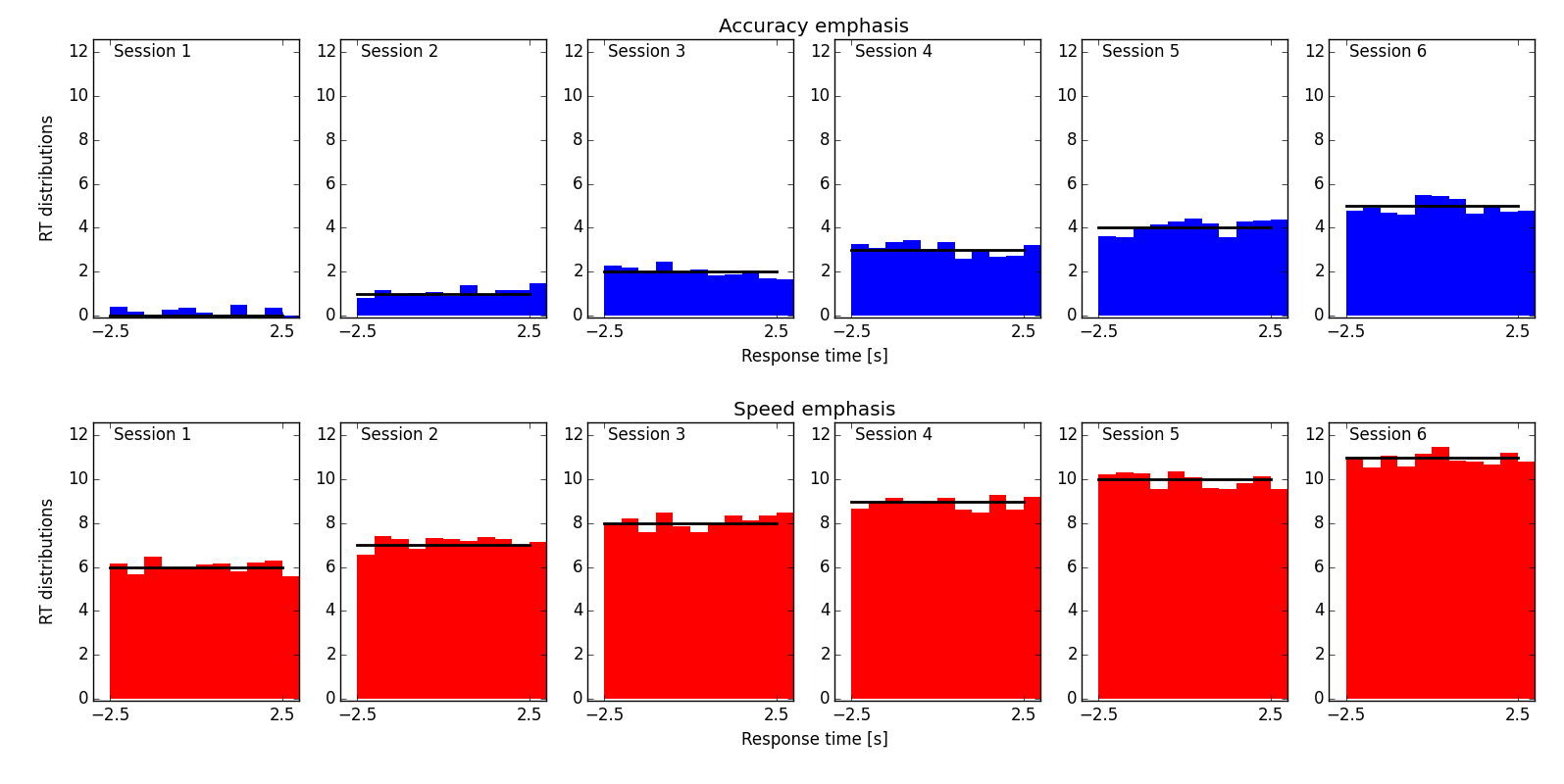# for Posterior predictive data distributions

Hi, Experts,

My colleagues and I have a question, how we can make a plot via python like
below. According to a guy's original paper, "Each panel shows the
normalized histograms of the observed data (bar plots) and the model
prediction (black lines) ".

I believe that people can make it with Matplotlib. Any code suggestion
(with simple example data) would be much appreciated.

(I am more comfortable with Matlab, but now the python code is preferred).

J

Juan,

If you join the mailing list you will be able to post without moderation.

Those look like a combination of `ax.hist` or `ax.bar` + `ax.plot(x, y, lw=3, c='k')` + `fig, ax_list = plt.subplots(1, 6)` + turning off some of the spines + `fig.text` for the shared axes labels and `fig.suptitle` for the titles.

Tom

···

Hi, Experts,

My colleagues and I have a question, how we can make a plot via python like below. According to a guy’s original paper, "Each panel shows the normalized histograms of the observed data (bar plots) and the model prediction (black lines) ".

I believe that people can make it with Matplotlib. Any code suggestion (with simple example data) would be much appreciated.

(I am more comfortable with Matlab, but now the python code is preferred).

J

Juan,

It is, of course, very difficult to give any concrete advice without knowing how your data are stored.

In any case, seaborn builds on matplotlib to provide some very advanced visualization through very concise code.

I recommend you look into the seaborn.distplot function and seaborn,FacetGrid class.

-Paul···

On Fri, Jun 5, 2015 at 9:14 AM, Juan Wu <wujuan22@…287…> wrote:

Matplotlib-users mailing list

Matplotlib-users@lists.sourceforge.net

https://lists.sourceforge.net/lists/listinfo/matplotlib-users

Hi, Experts,

My colleagues and I have a question, how we can make a plot via python like below. According to a guy’s original paper, "Each panel shows the normalized histograms of the observed data (bar plots) and the model prediction (black lines) ".

I believe that people can make it with Matplotlib. Any code suggestion (with simple example data) would be much appreciated.

(I am more comfortable with Matlab, but now the python code is preferred).

J

(apologies if the list receives this twice)

···

Juan,

It is, of course, very difficult to give any concrete advice without knowing how your data are stored.

In any case, seaborn builds on matplotlib to provide some very advanced visualization with a very concise API.

I recommend you look into the seaborn.distplot function and seaborn.FacetGrid class.

-Paul

On Fri, Jun 5, 2015 at 9:14 AM, Juan Wu <wujuan22@…287…> wrote:

Hi, Experts,

My colleagues and I have a question, how we can make a plot via python like below. According to a guy’s original paper, "Each panel shows the normalized histograms of the observed data (bar plots) and the model prediction (black lines) ".

I believe that people can make it with Matplotlib. Any code suggestion (with simple example data) would be much appreciated.

(I am more comfortable with Matlab, but now the python code is preferred).

J

I put the data into a list of lists of numpy arrays. The following script generated a plot similar to what Juan attached:

import matplotlib.pyplot as plt
import numpy as np

def model(t, ii, jj):
“”"
Returns some numbers according to the independent variable, t, and
parameters of the model, ii and jj.
“”"
return (jj*6+ii)*np.ones_like(t)

### generate data (i.e. model + some random values)

t = np.linspace(-2.5, 2.5, 11)
data = [
[model(t, ii, jj) + np.random.rand(len(t)) - .5
for ii in range(6)] # different sessions
for jj in range(2)] # accuracy/speed

### do the plotting

colors = [‘b’, ‘r’]
titles = [‘Accuracy emphasis’, ‘Speed emphasis’]
fig, big_axes = plt.subplots(figsize=(16, 6), nrows=2, ncols=1, sharey=True)

for row, big_ax in enumerate(big_axes):
big_ax.set_ylabel(‘RT distributions’)
big_ax.set_xlabel(‘Response time [s]’)
big_ax.set_title(titles[row])
big_ax.tick_params(labelcolor=(1.,1.,1.,0.), top=‘off’, bottom=‘off’, left=‘off’, right=‘off’)
big_ax._frameon = False

for jj, emph_data in enumerate(data):
for ii, iidata in enumerate(emph_data):
# plot bars:
ax.bar(t, iidata,
color=colors[jj],
width=(max(t)-min(t))/(len(t)-1), # for non-overlapping bars
linewidth=0) # no outlines
# plot models:
ax.plot(t, model(t, ii, jj), ‘k’, linewidth=2)

``````    # annotate axes etc.
ax.set_xticks((-2.5, 0., 2.5))
ax.set_ylim((-.1, 12.8))
ax.annotate('Session {}'.format(ii+1), xy=(-2.4, 11.8))
``````

plt.tight_layout()
plt.show()

How to add the centered titles for each row is described here : http://stackoverflow.com/questions/27426668/row-titles-for-matplotlib-subplot

Jan···

On 8 June 2015 at 03:27, Paul Hobson <pmhobson@…287…> wrote:

(apologies if the list receives this twice)

Matplotlib-users mailing list

Matplotlib-users@lists.sourceforge.net

https://lists.sourceforge.net/lists/listinfo/matplotlib-users

Juan,

It is, of course, very difficult to give any concrete advice without knowing how your data are stored.

In any case, seaborn builds on matplotlib to provide some very advanced visualization with a very concise API.

I recommend you look into the seaborn.distplot function and seaborn.FacetGrid class.

-Paul

On Fri, Jun 5, 2015 at 9:14 AM, Juan Wu <wujuan22@…287…> wrote:

Hi, Experts,

My colleagues and I have a question, how we can make a plot via python like below. According to a guy’s original paper, "Each panel shows the normalized histograms of the observed data (bar plots) and the model prediction (black lines) ".

I believe that people can make it with Matplotlib. Any code suggestion (with simple example data) would be much appreciated.

(I am more comfortable with Matlab, but now the python code is preferred).

J